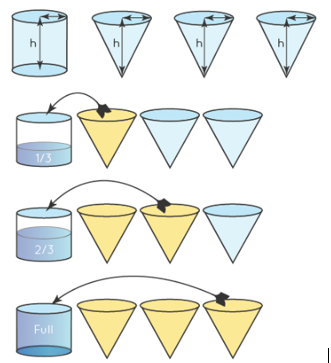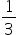Maths-
General
Easy

Question

# Ratio of volume of a cone to the volume of a cylinder for same base radius andsame height is __________

## 3:11:32:11:2Hint:

## The correct answer is: 1:3

### Let us take a cylinder  of height "h", base radius "r", and take 3 cones of height "h". Fill the cones with water and empty out one cone at a timeEach cone fills the cylinder to one-third quantity. Hence, such three cones will fill the cylinder. Thus, the volume of a cone is one-third of the volume of the cylinder. Volume of cone = (1/3) × Volume of cylinder= () × πr2h= ()πr2hSo the ratio of volume of cone to the volume of cylinder is 1:3Therefore , the correct option is b) 1:3#### With Turito Foundation.#### Get an Expert Advice From Turito.